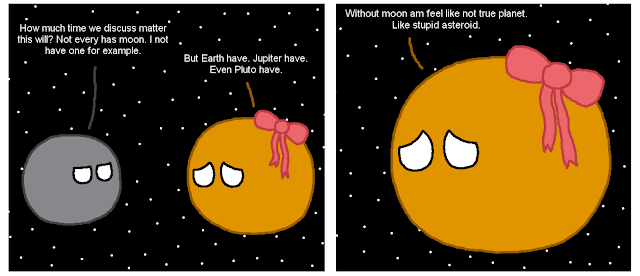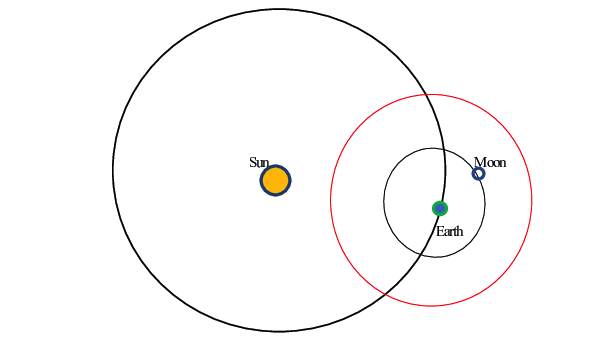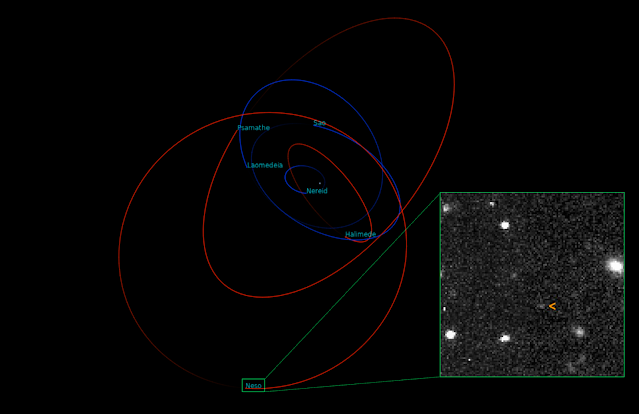### Hill Spheres: Where the MoonMoons Are

"We are, many of us, a planet orbiting somebody's sun, unconscious of a lonely moon, orbiting our planet" - Robert Breault

_____________________________________

In case Astronomical Returns hadn't made it obvious already, 4-year-old Hans was little space nerd who tried to memorize every conceivable fact about each of the 9 planets (yes, there were 9 planets until I turned ten). Naturally I knew exactly how many moons each planet had, and it always struck me as odd that Mercury and Venus didn't have any, when even little ol' Pluto had a cosmic companion. Of course, orbital mechanics meant nothing to me back then, I had no idea why that was the case; I assumed it was just coincidence, as if Mercury and Venus were the two weirdos that nobody wanted to take to the school dance.Poor Venus | From Reddit r/planetball

If you were to take an educated guess, you might surmise it has to do with the two planets' proximity to the Sun. But let's table that for now, we'll come back to it...

In orbital mechanics, the Hill Sphere of an astronomical body (named after American astronomer George William Hill) refers to its sphere of gravitational dominance, particularly in the face of perturbations (i.e. gravitational distortions) from the presence of a larger body. For example, Earth (the smaller body) orbits the Sun (a larger body): if you were to try put a satellite into low Earth orbit, the satellite should have no problem because the Earth's gravity is dominant there. But if you try put it into a super high Earth orbit, the satellite's orbit would be very unstable because of constant perturbations from the Sun; eventually, the satellite would break free from Earth's orbit and orbit the Sun instead. In that case, the satellite was trying to orbit outside of Earth's Hill Sphere, and was really in the Sun's!The red circle represent's Earth's Hill Sphere. Anything within it is inside of Earth's gravitational sphere of influence. Hence why the Moon orbits Earth and not the Sun

So how do we know how far Earth's Hill Sphere extends? The radius of any Hill Sphere can be approximated by the formula:

$r_H \approx a(1-e) \sqrt \frac{m}{3M}$        (see derivation here)

Whereby

• $a$: semi-major axis of the orbiting body (Earth)
• $e$: eccentricity of the orbiting body (Earth)
• $m$: mass of the orbiting body (Earth)
• $M$: mass of the central body (Sun)

Because Earth's orbit is basically circular (e = 0), the 1 - e term falls away from the formula (circular orbits are most favorable for orbital stability). If you crunch the numbers, you'll get that Earth's Hill Sphere extends 1.5M km away. For context, the Moon's orbit is 384,000 km away, proving that the Moon is comfortably within Earth's gravitational influence and won't get pulled away by the Sun into a heliocentric orbit.

And interestingly, if you think of Hill Spheres in the context of Kepler's Third Law of Planetary Motion (which relates orbital period to the semi-major axis of the orbit), that means that the longest possible orbital period of an Earth satellite is seven months. If you tried to have a satellite orbit more slowly, you'd have to raise its orbit beyond Earth's Hill Sphere, and it would eventually end up orbiting the Sun!

$T = 2\pi \sqrt \frac{a^3}{GM}$

• $G$: gravitational constant
• $M$: mass of the central body (Earth in this case)

Back to the question about Mercury and Venus' lack of moons - the above table applies the same formula to determine the Hill Spheres of each of the 8 planets. Immediately you'll see Mercury would have a tough (but not impossible) time holding onto a moon, as its Hill Sphere is less than 1/8th the size of Earth's. Venus' chances were somewhat better with a Hill Sphere about 2/3rds of Earth's, but alas it never got anything. Our moon came about after primordial Earth suffered a planetary-scale collision with another object, so there was a ton of debris within Earth's Hill Sphere that easily coalesced into a moon. As for Mars, well, I guess Mars was just luckier than Venus; their Hill Spheres are about the same, but Mars happened to get Phobos and Deimos.

It's clear the size of a body's Hill Sphere is a factor of both its distance from the larger body (semi-major axis) and its mass. The question is, which matters more? The answer is the distance, and this is apparent in the formula: you'll notice the ratio of masses (i.e. the Earth's mass vs the Sun's mass) is under the cube root function, so it has much less of an impact on the Hill Sphere than the semi-major axis. For this reason, Pluto's Hill Sphere is 4x bigger than Earth's. Its distance from the Sun (the perturbing body) matters way more than its mass. As it turns out, Neptune has the biggest Hill Sphere in the solar system because it has the best combination of mass and distance from the Sun.Neptune's enormous Hill Sphere allows for some really wacky satellites. Its most distant moon, Neso, orbits about 130x further than our moon orbits Earth, and it takes over 26 years to orbit Neptune

Lastly, there's one more interesting hypothetical question I came across in researching Hill Spheres: could an astronaut have been able to orbit a Space Shuttle placed in low Earth orbit? The Space Shuttle weighed about 104 tons and orbited 300 km above the Earth, so if you plug those assumptions into the formula, you'd get that its Hill Sphere in LEO would be a measly 120 cm, way smaller than the shuttle's own volume! In order to compress the 104 tons into that 120 cm sphere, the Space Shuttle would have needed the density of a ball of solid lead!

I liked this thought experiment because it allows us to relate Hill Spheres to satellite densities. Turns out it's not so easy to create a moonmoon!